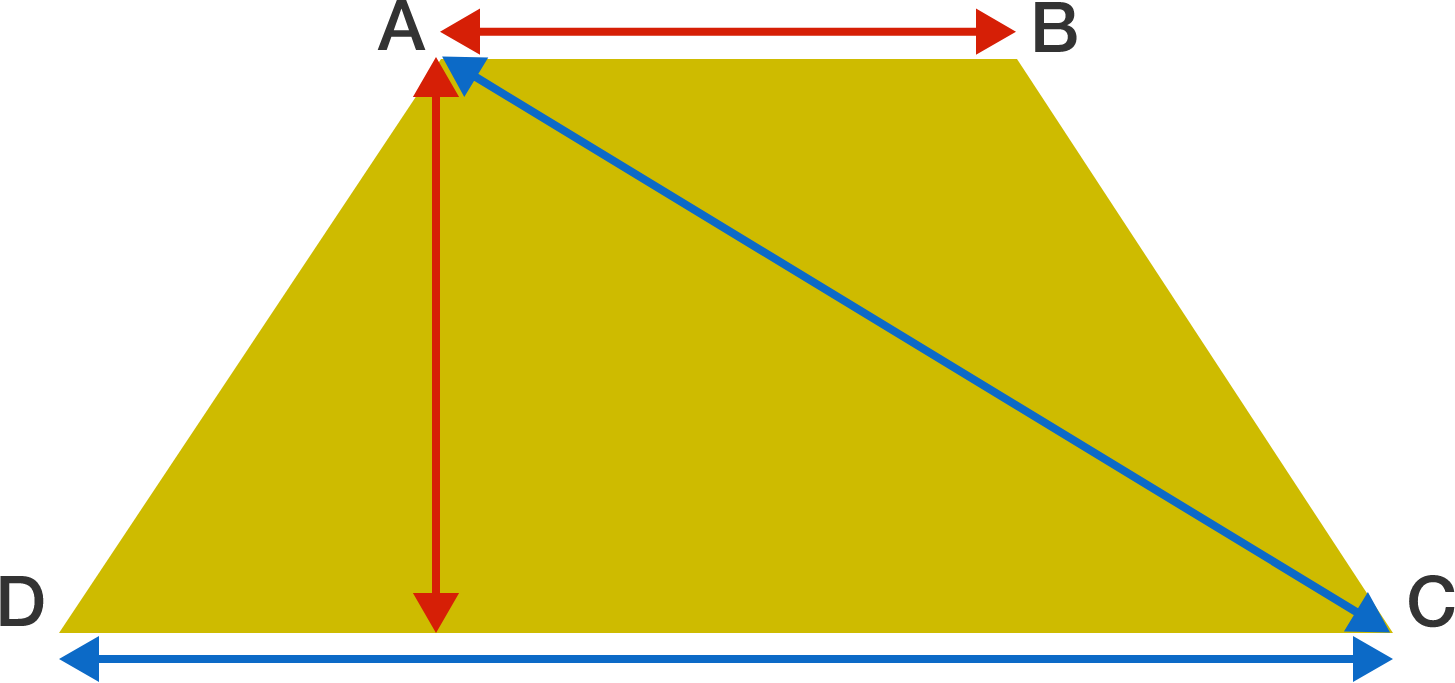# Don't get trapped

Geometry Level 2Suppose $ABCD$ is an isosceles trapezoid with bases $AB$ and $CD$ and sides $AD$ and $BC$ such that $|CD| \gt |AB|.$ Also suppose that $|CD| = |AC|$ and that the altitude of the trapezoid is equal to $|AB|.$

If $\dfrac{|AB|}{|CD|} = \dfrac{a}{b},$ where $a$ and $b$ are positive coprime integers, then find $\large a^{b}.$

×

Problem Loading...

Note Loading...

Set Loading...